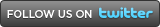A WARBIRDS RESOURCE
GROUP WEBSITE

DEPARTMENTS:

Custom SearchSTAFF
PX STORE
SUPPORT THE SITE

WB550  (D-07)
WB563  (A-07)
WB567  (A-16)
WB569  (A-07)
WB585  (A-07)
WB588  (A-04)
WB619  (A-07)
WB621  (A-08)
WB668  (A-08)
WB684  (A-16)
WB703  (A-16)
WB710  (A-16)
WB726  (A-07)
WB733  (D-07)
WB750  (A-06)
WD287  (A-16)
WD310  (A-07)
WD373  (A-07)
WG357  (A-08)
WG472  (A-07)
WG474  (A-07)
WG478  (A-08)
WG480  (A-12)
WG482  (?-18)
WK507  (A-15)
WK550  (A-11)
WK558  (A-16)
WK611  (A-07)
WK630  (A-06)
WK635  (S-06)
WP790  (D-06)
WP859  (A-07)
WP912  (D-07)
WP962  (D-07)
WP981  (A-08)WOULD YOU LIKE
TO SUPPORT
THIS SITE?